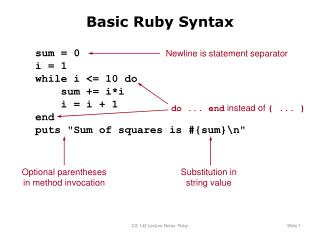Download PresentationBasic Ruby Syntax

Basic Ruby Syntax - PowerPoint PPT Presentation

Basic Ruby Syntax. sum = 0 i = 1 while i <= 10 do sum += i*i i = i + 1 end puts "Sum of squares is #{sum}
". Newline is statement separator. do ... end instead of { ... }. Optional parentheses in method invocation. Substitution in string value. Variable Names and Scopes.I am the owner, or an agent authorized to act on behalf of the owner, of the copyrighted work described.
Download PresentationBasic Ruby Syntax

Download Policy: Content on the Website is provided to you AS IS for your information and personal use and may not be sold / licensed / shared on other websites without getting consent from its author.While downloading, if for some reason you are not able to download a presentation, the publisher may have deleted the file from their server.

- - - - - - - - - - - - - - - - - - - - - - - - - - E N D - - - - - - - - - - - - - - - - - - - - - - - - - -
Presentation Transcript
1. Basic Ruby Syntax sum = 0 i = 1 while i <= 10 do sum += i*i i = i + 1 end puts "Sum of squares is #{sum}\n" Newline is statement separator do ... end instead of { ... } Optional parentheses in method invocation Substitution instring value CS 142 Lecture Notes: Ruby

2. Variable Names and Scopes foo Local variable \$foo Global variable @foo Instance variable in object @@foo Class variable MAX_USERS Constant (by convention) CS 142 Lecture Notes: Ruby

3. Ruby Statements if x < 10 then ... elsif x < 20 ... else ... end while x < 10 do ... end array = [14, 22, 34, 46, 92] for value in array do ... end CS 142 Lecture Notes: Ruby

4. Factorial def fac(x) if x <= 0 then return 1 end return x*fac(x-1) end CS 142 Lecture Notes: Ruby

5. Arguments: Defaults, Variable # def inc(value, amount=1) value+amount end def max(first, *rest) max = first for x in rest do| if (x > max) then max = x end end return max end CS 142 Lecture Notes: Ruby

6. Keyword Arguments def create_widget(size, properties) ... end create_widget(6, {:id => "table22", :class => "Cart"}) create_widget(6, :id => "table22", :class => "Cart") CS 142 Lecture Notes: Ruby

7. Blocks, Iterators, Yield oddNumbers(3) do |i| print(i, "\n") end def oddNumbers(count) number = 1 while count > 0 do yield(number) number += 2 count -= 1 end end Block: code passedto method Invoke method’s block CS 142 Lecture Notes: Ruby

8. Another Block/Iterator Example def sumOdd(count) sum = 0 oddNumbers(count) do |i| sum += i end return sum end def oddNumbers(count) number = 1 while count > 0 do yield(number) number += 2 count -= 1 end end CS 142 Lecture Notes: Ruby

9. Equivalent Code array = [14, 22, 34, 46, 92] for value in array do print(value, "\n") end array = [14, 22, 34, 46, 92]; array.each do |value| print(value, "\n") end CS 142 Lecture Notes: Ruby

10. Simple Class class Point def initialize(x, y) @x = x @y = y end def x @x end def x=(value) @x = value end end p = Point.new(3,4) puts "p.x is #{p.x}\n" p.x = 44; CS 142 Lecture Notes: Ruby

11. Module Example New methods available in MyClass: min, max, sort, map, select, ... class MyClass include Enumerable ... def each ... end end CS 142 Lecture Notes: Ruby

12. CS 142 Lecture Notes: Ruby